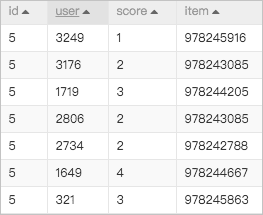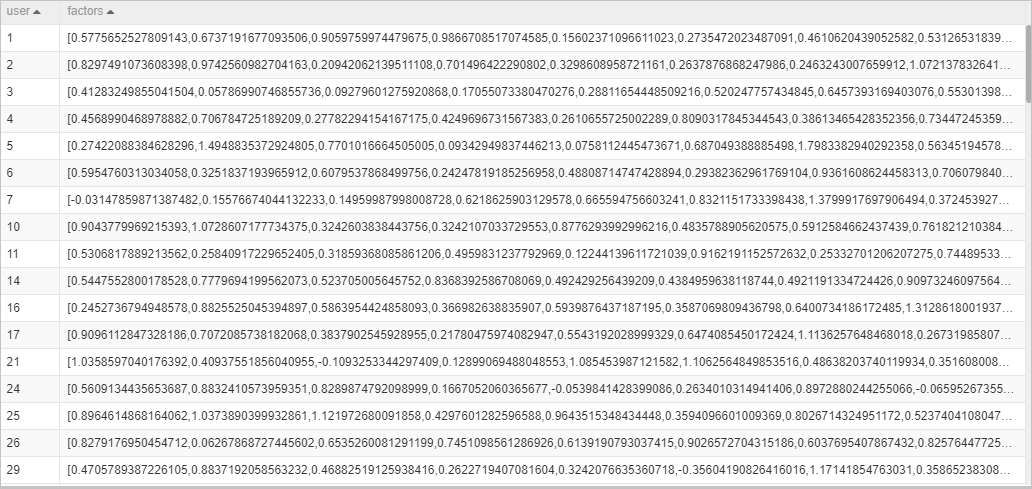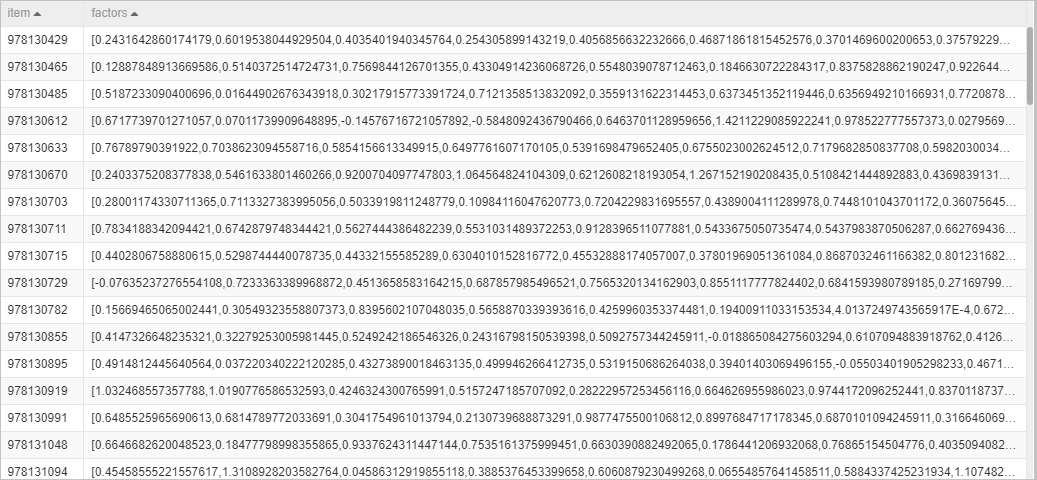Alternating Least Squares (ALS) is a model-based recommendation algorithm. It factorizes models by using sparse matrix factorization and predicts the values of missing entries to obtain a basic training model. ALS is also known as a hybrid collaborative filtering algorithm that combines users and items.

## Configure the component

Machine Learning Studio provides an ALS algorithm template. You can log on to the Machine Learning Studio for AI (PAI) console and switch back to the former version. On the page that appears, click Create in the ALS music recommendations section. Then, in the New Experiment dialog box, configure the parameters to create an ALS experiment. You can configure the component only in the PAI console.
Tab Parameter Description
Fields Setting User Column The name of the user ID column in the input table. Data in the column must be of the BIGINT type.
Item Column The name of the item column in the input table. Data in the column must be of the BIGINT type.
Score Column The name of the column that contains the scores provided by users for items in the input table. Only the columns of numeric data types are supported.
Parameters Setting Factors The number of factors. Valid values: (0,+∞). Default value: 10.
Iterations The number of iterations. Valid values: (0,+∞). Default value: 10.
Regularization Coefficient The regularization coefficient. Valid values: (0,+∞). Default value: 0.1.
Implicit Preference Model Specifies whether to use the implicit preference model.
Implicit Preference Coefficient The implicit preference coefficient. Valid values: (0,+∞). Default value: 40.
Tuning Cores The number of cores. Valid values: 1 to 9999.
Node Memory The memory size of each core. Valid values: 1024 to 64 × 1024. Unit: MB.
If you use the following data as input for the ALS algorithm template, you can obtain the following Matrix X and Matrix Y.
• Input data• Matrix X• Matrix YIf you want to predict the score of the 994556636 item provided by User 1, multiply Vector X and Vector Y.
``````# Vector X
[-0.14220297,0.8327106,0.5352268,0.6336995,1.2326205,0.7112976,0.9794858,0.8489773,0.330319,0.7426911]
# Vector Y
[0.71699333,0.5847747,0.96564907,0.36637592,0.77271074,0.52454436,0.69028413,0.2341857,0.73444265,0.8352135]``````